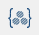# Define Stress versus Strain Curve

Define a stress versus strain curve that is referenced by a nonlinear material property.

SimSolid assumes an engineering stress-strain curve.
Engineering stress
Assumes a constant non-changing cross-section area during deformation.
True Stress
Assumes the cross-section area that changes at every instant during deformation.

Some sources use true stress-strain curves. They can be imported by SimSolid from a CSV file.

1. In the Project Tree, click on the Assembly branch to open the Assembly workbench.
2. On the workbench toolbar, click the(Apply materials) icon.
3. In the dialog, choose a material to activate the Stress-strain curve button.
4. Click the Stress-strain curve button.
5. In the Stress-strain curve dialog, use one of the following methods to define a stress-strain curve:
Method Process
Import from CSV
1. Click the Import CSV button.
2. In the File browser, choose the desired CSV and click Open.
Create from N-values Create stress-strain curve using a K-n power law function.
• Click the Create by n-value button.
• Specify K and n.
Some sample K-n values for typical materials are given in the drop-down menu.
Create manually
• Select the Add row button.
• Enter the stress and strain values. At minimum, 3 points are required.
The results will be plotted in the XY graph in the dialog. You can use the scroll wheel to zoom in, the left mouse button to pan, or the Refit button to reset the graph.
6. Optional: Click the Edit elasticity modulus button to update the elasticity modulus of a selected material.
The stress-strain curve is automatically updated to reflect the elasticity modulus entered.
If the elasticity modulus defined is less than the elasticity modulus derived from the curve, the stress-strain curve is trimmed by intersecting the line that defines the new elasticity modulus and the stress-strain curve.
Note: If an x-value input is outside the range of x-values specified on the Table, the corresponding y-value look up is performed using linear extrapolation from the two end points.